﻿ 基于CFD和热阻网络法的C型舱LEG船温度场分析及热应力计算
 舰船科学技术2023, Vol. 45Issue (1): 18-23    DOI: 10.3404/j.issn.1672-7649.2023.01.004PDF

Temperature field analysis of LEG carrier of tank type-C based on CFD/thermal resistor network method and thermal stress calculation
WANG Wei-fei, YANG Xue-hang, HAN Yu, ZHANG Wei
Marine Design and Research Institute of China, Shanghai 200011, China
Abstract: When performing temperature field calculation for LEG carrier of tank type-C, the CFD method cannot take the structural detail into account and the thermal resistor network method cannot consider the convective thermal exchange accurately enough. In order to solve the drawbacks, a new method is proposed by combining the two preceding methods to calculate the temperature field of hull structure. During the CFD analysis, only primary structure members are considered, and the results are utilized as inputs for thermal resistor network method analysis, where the structural details are considered. Calculation results indicate that the proposed method is effective and reliable, which can avoid the drawbacks of the two methods and suitable for temperature field calculation for hull structure. Thus, the proposed method is appropriate for engineering practice. Results also shows the thermal stress shall be considered for structure strength assessment for structure regions bear significant temperature gradient.
Key words: LEG carrier     temperature field     CFD method     thermal resistor network method     thermal stress
0 引　言

C型舱由于方案成熟、可靠性高、成本较低等优点，在中小型LEG/LNG运输船上广泛采用，并在近几年逐步作为船上的液化气燃料舱。对于装载温度极低货品的C型舱，需要根据规范要求[1-2]，按照船体结构温度场分布，选取船体结构的材料钢级。对于一些非常规的液舱布置形式，在进行结构强度评估时需要考虑温度载荷导致的热应力。

1 基本原理与方法

1.1 热传导

 $q=\lambda \Delta T。$ (1)

1.2 热对流

 $q=h({T}_{s}-{T}_{f})。$ (2)

1.3 热辐射

 $q=\varepsilon \sigma \left({T}_{1}^{4}-{T}_{2}^{4}\right) 。$ (3)

1.4 有限体积法热学计算

 $\frac{\partial \rho }{\partial t}+\frac{\partial \left(\rho {U}_{j}\right)}{\partial {x}_{j}}=0，$ (4)

 $\begin{split}& \frac{\partial \left(\rho {U}_{j}\right)}{\partial t}+\frac{\partial \left(\rho {{U}_{i}U}_{j}\right)}{\partial {x}_{i}}= \\ &-\frac{\partial P}{\partial {x}_{j}}+\frac{\partial }{\partial {x}_{i}}\left(\mu \left(\frac{\partial {U}_{i}}{\partial {x}_{j}}+\frac{\partial {U}_{j}}{\partial {x}_{i}}\right)-\overline{\rho {u}_{i}{u}_{j}}\right)+{F}_{{U}_{j}} ，\end{split}$ (5)

 $\begin{split} &\dfrac{\partial \left[\rho \left(e+\dfrac{{U}^{2}}{2}\right)\right]}{\partial t}+\dfrac{\partial \left[\rho {U}_{i}\left(e+\dfrac{{U}^{2}}{2}\right)\right]}{\partial {x}_{i}}=\\ &-\dfrac{\partial {q}_{i}}{\partial {x}_{i}}-\dfrac{\partial {PU}_{i}}{\partial {x}_{i}}-\dfrac{\partial }{\partial {x}_{i}}\left[{U}_{i}\mu \left(\dfrac{\partial {U}_{i}}{\partial {x}_{j}}+\dfrac{\partial {U}_{j}}{\partial {x}_{i}}\right)\right]+{q}'。\end{split}$ (6)

1.5 热阻网络法热学计算

 $\sum {Q}_{i} =0 ，$ (7)

2 计算参数和模型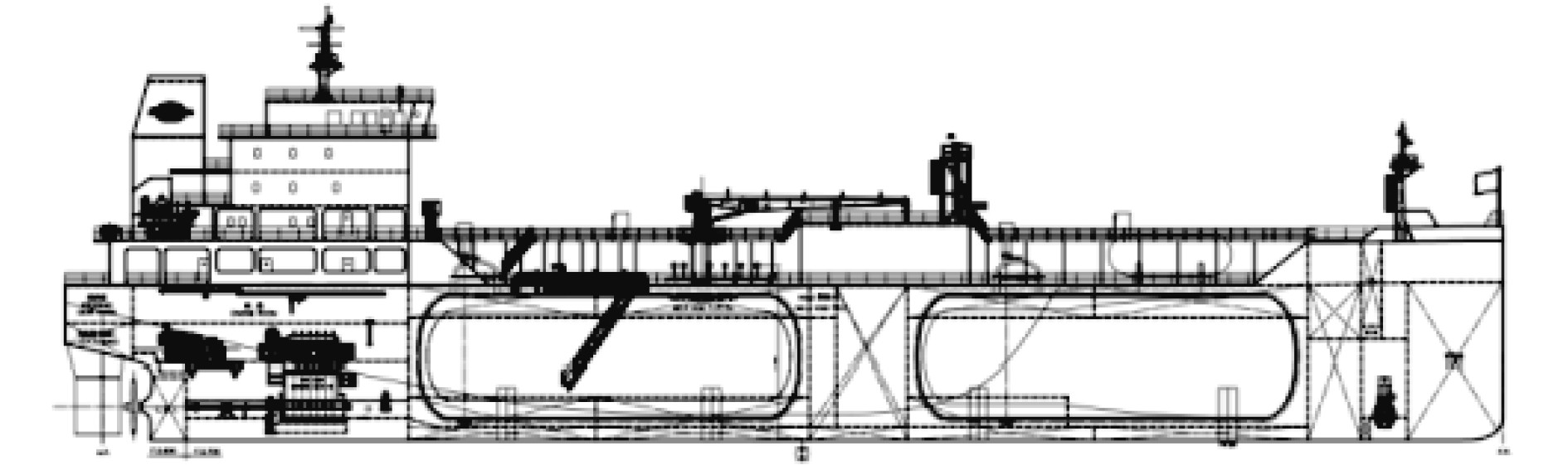图 1 6 500 m3LEG船总布置 Fig. 1 General arrangement of 6 500 m3 LEG carrier
2.1 计算参数表 1 环境条件 Tab.1 Environment condition表 2 材料的物理特性参数 Tab.2 Physical properties of material

CFD方法与热阻网络法的边界条件一致，按船体结构定义如下：

2.2 CFD方法计算模型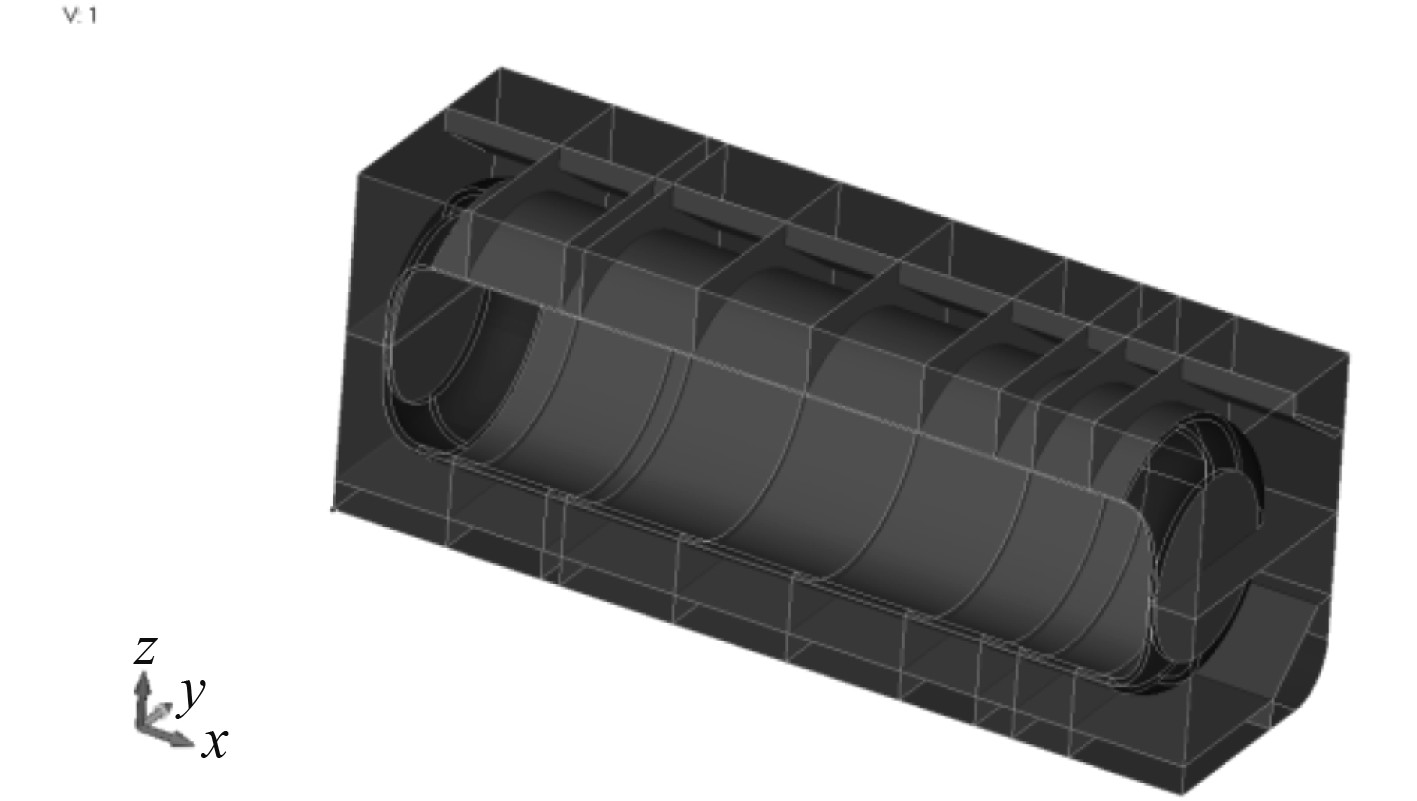图 2 CFD计算几何模型 Fig. 2 Geometry model for CFD calculation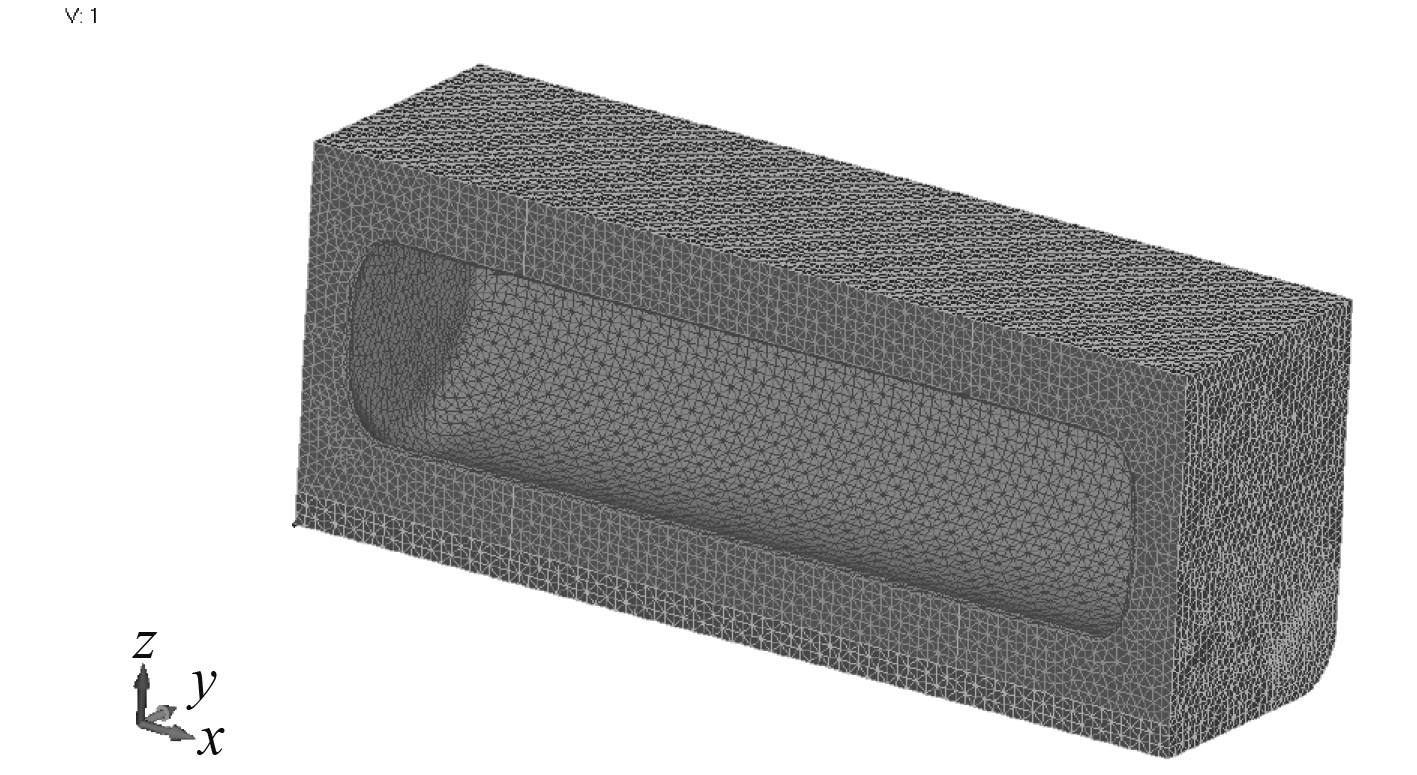图 3 CFD计算网格模型 Fig. 3 Mesh model for CFD calculation

2.3 热阻网络法计算模型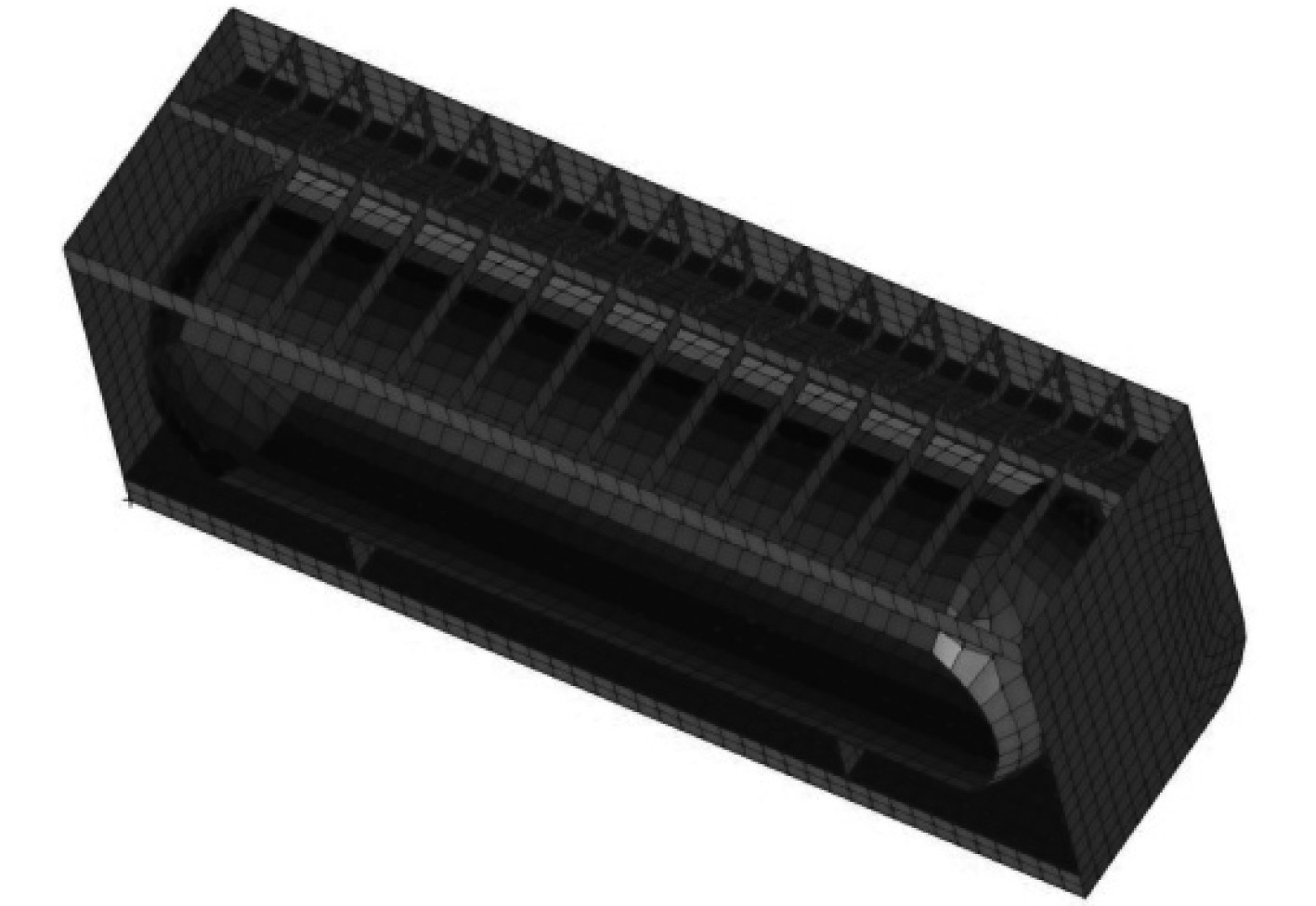图 4 热阻网络法模型 Fig. 4 Model for thermal resistor network method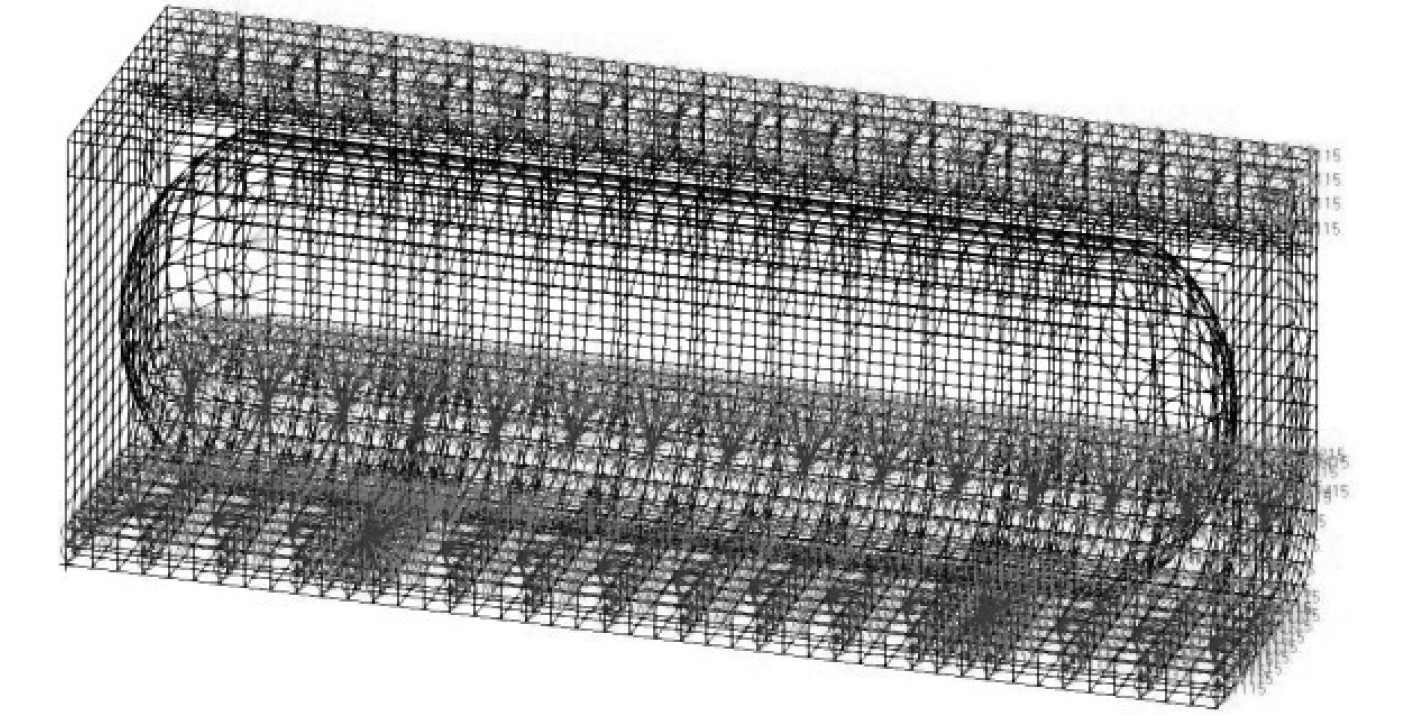图 5 热阻网络法热对流空间定义 Fig. 5 Definition of convection space for thermal resistor network method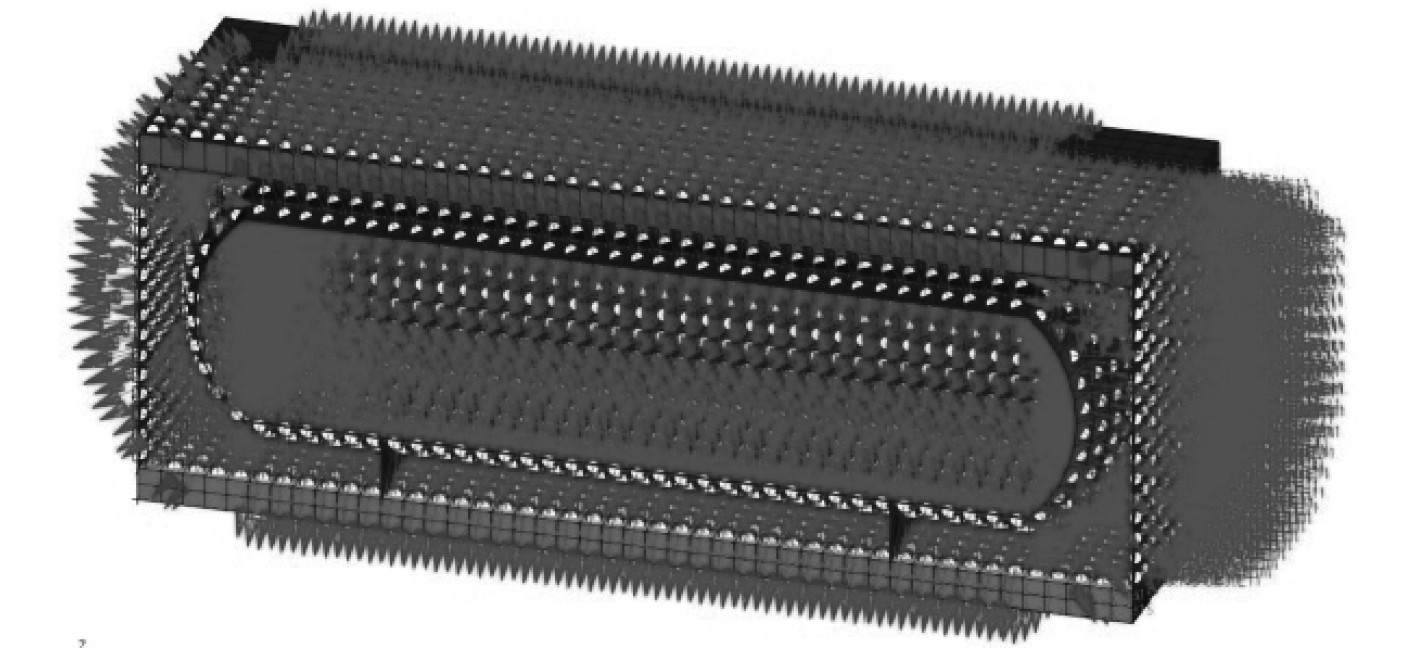图 6 C型舱与船体辐射空间的定义 Fig. 6 Radiation enclosure definition between type C tank and hull
3 温度场计算结果 3.1 CFD方法计算结果

CFD方法计算得到的货舱区域的流场速度矢量横剖面分布如图7所示。从流场速度分布可以看出，对于目标船型，由于对流引起的流体流速较低，且在双层底区域的底压载舱，流速非常均匀。舱段模型中，空气和水的温度分布如图8图11所示。从图8可以看出，在2个鞍座中间区域，由于鞍座结构对流场的阻挡，该区域流场的温度明显低于货舱两端的温度。简化方法由于其局限性，无法得到该温度分布。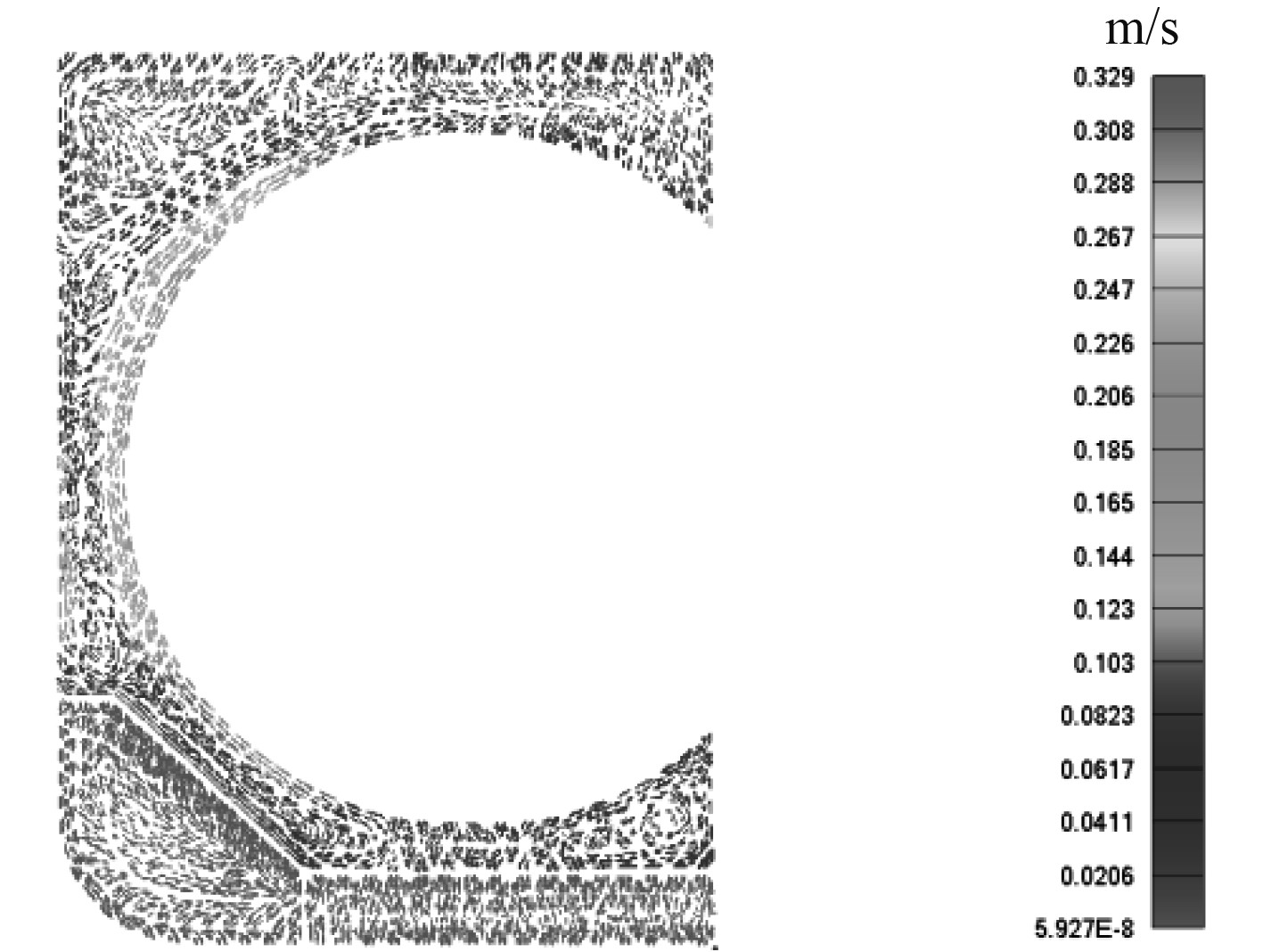图 7 流体速度矢量 Fig. 7 Flow velocity vector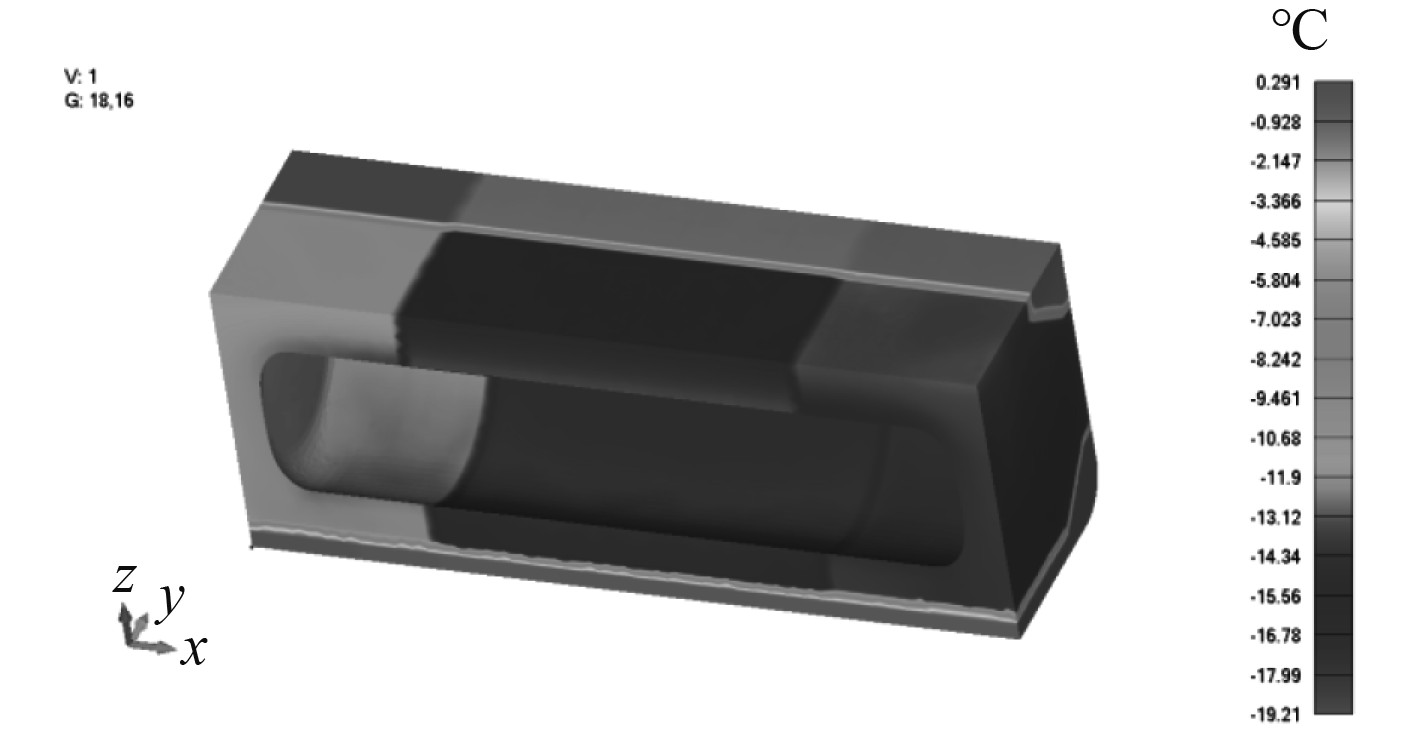图 8 货舱段流体温度分布 Fig. 8 Flow temperature distribution of cargo hold area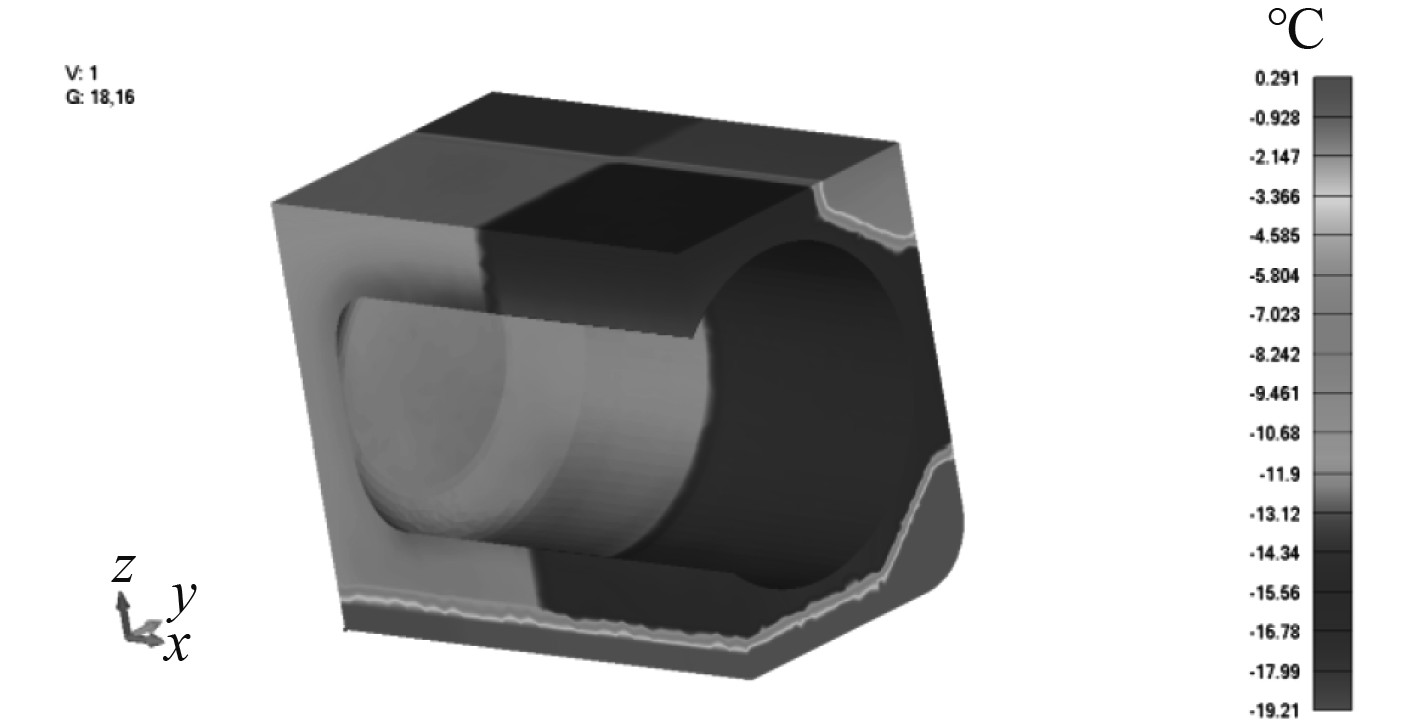图 9 典型横剖面流场温度分布 Fig. 9 Flow temperature distribution of typical transverse section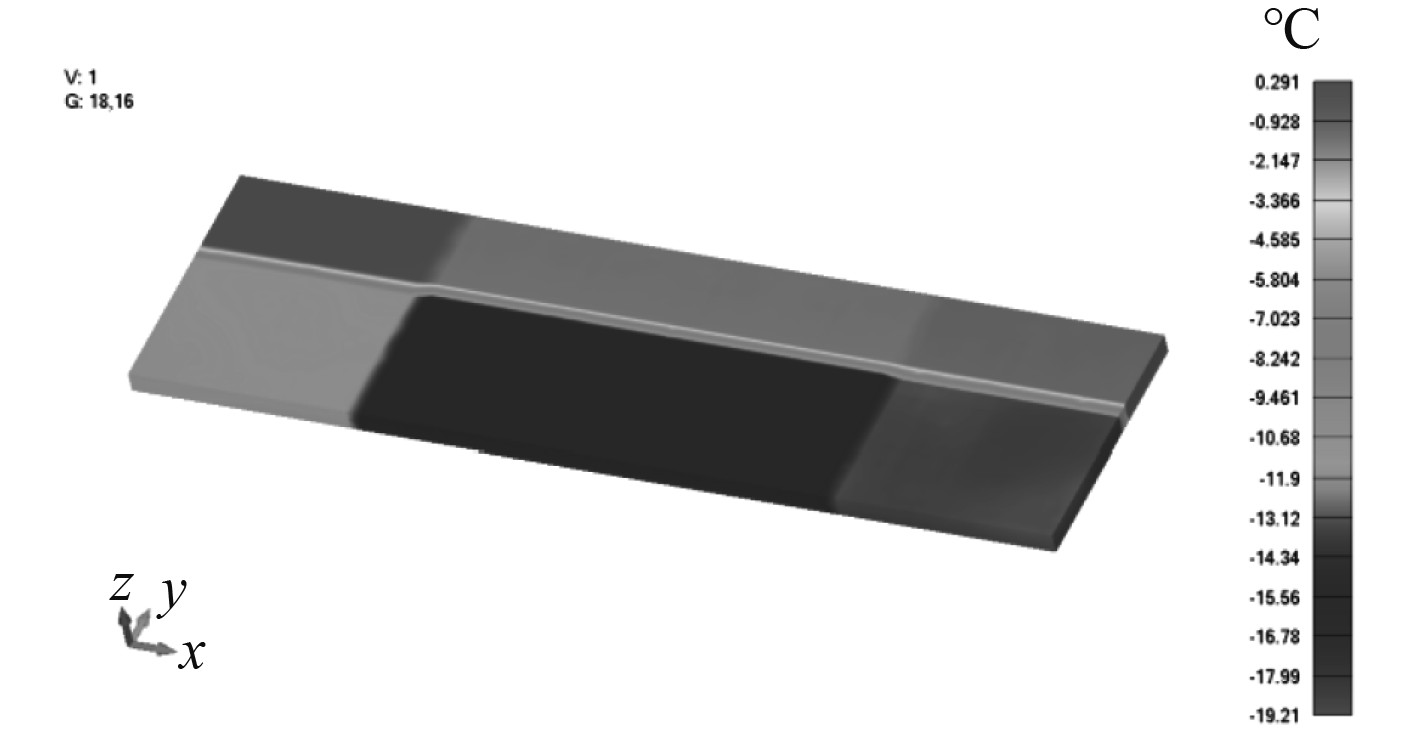图 10 上甲板区域流体温度分布 Fig. 10 Flow temperature distribution of upper deck region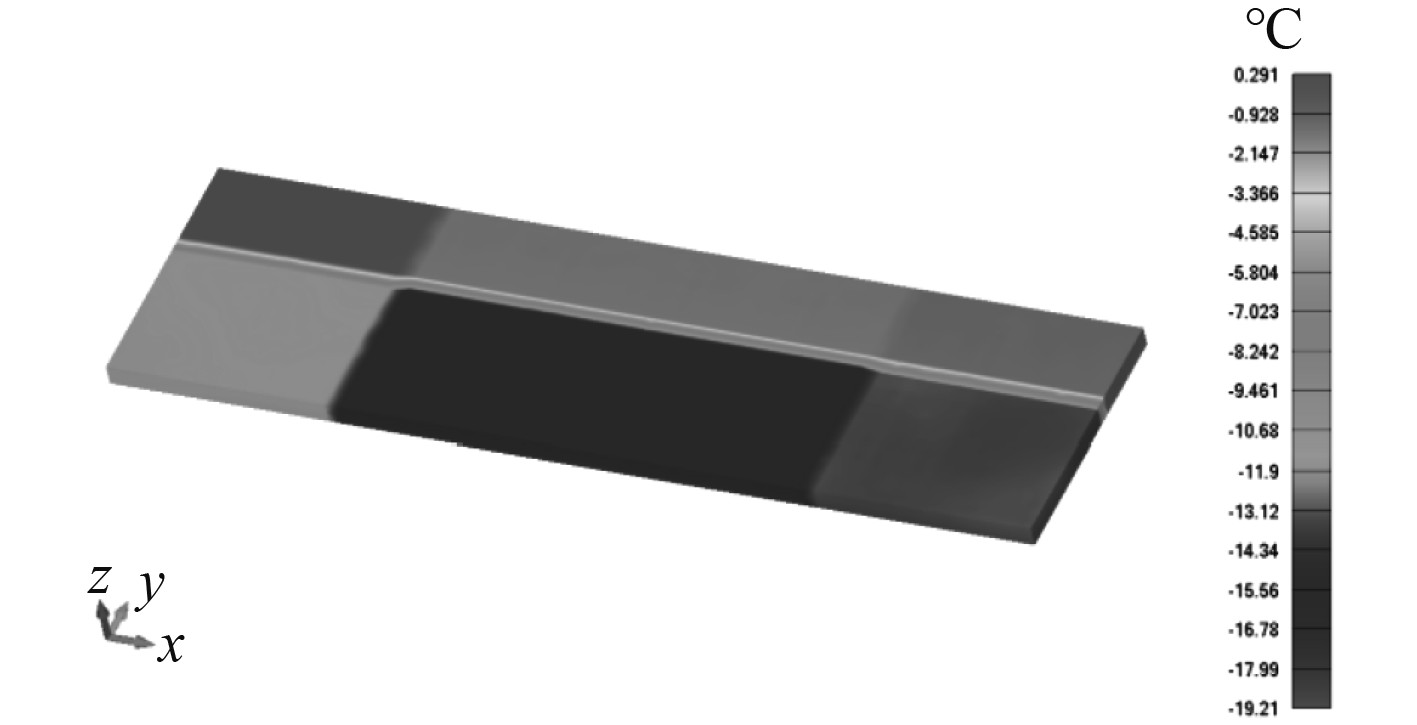图 11 底部区域流体温度分布 Fig. 11 Flow temperature distribution of bottom region

CFD方法计算得到的船体结构温度场如图12图15所示。由于材料的导热性差异，可以看到船体结构的温度梯度比相关区域流场的温度梯度小的多。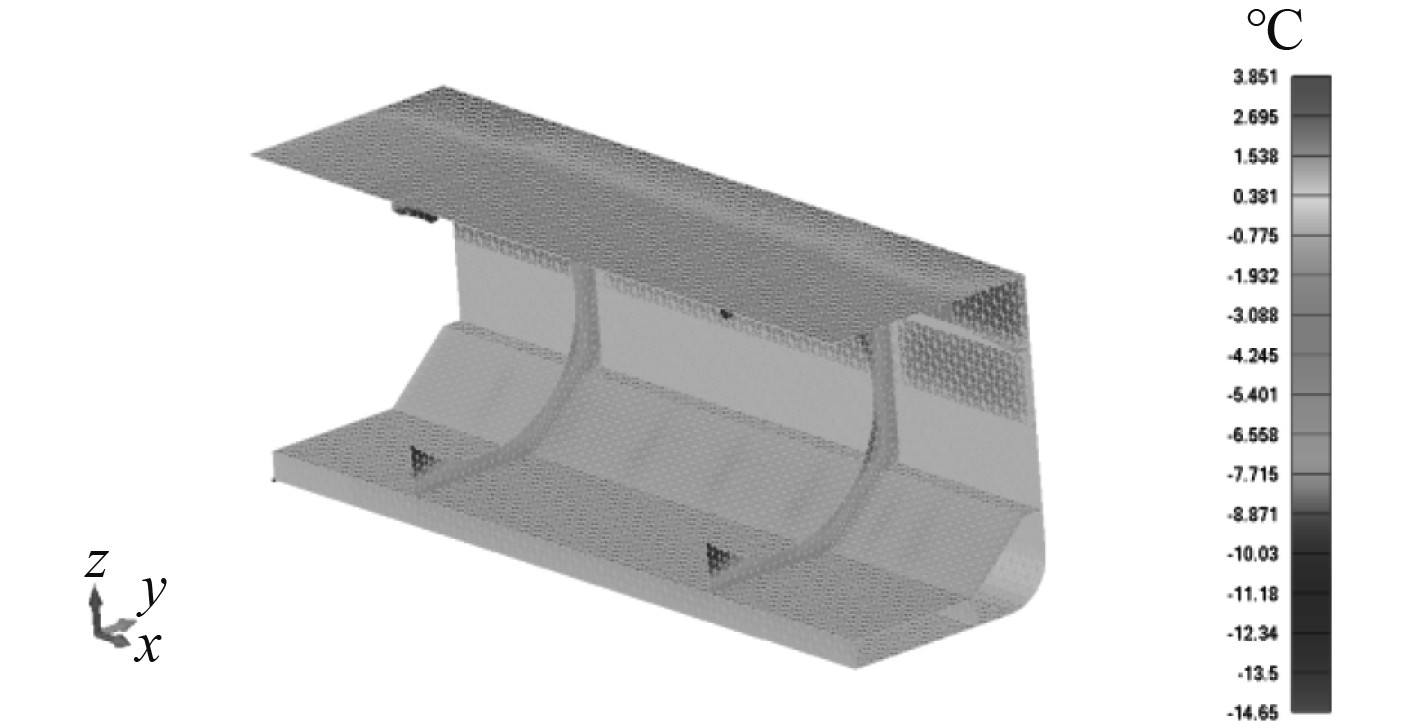图 12 舱段结构温度场 Fig. 12 Hull structure temperature field of cargo hold area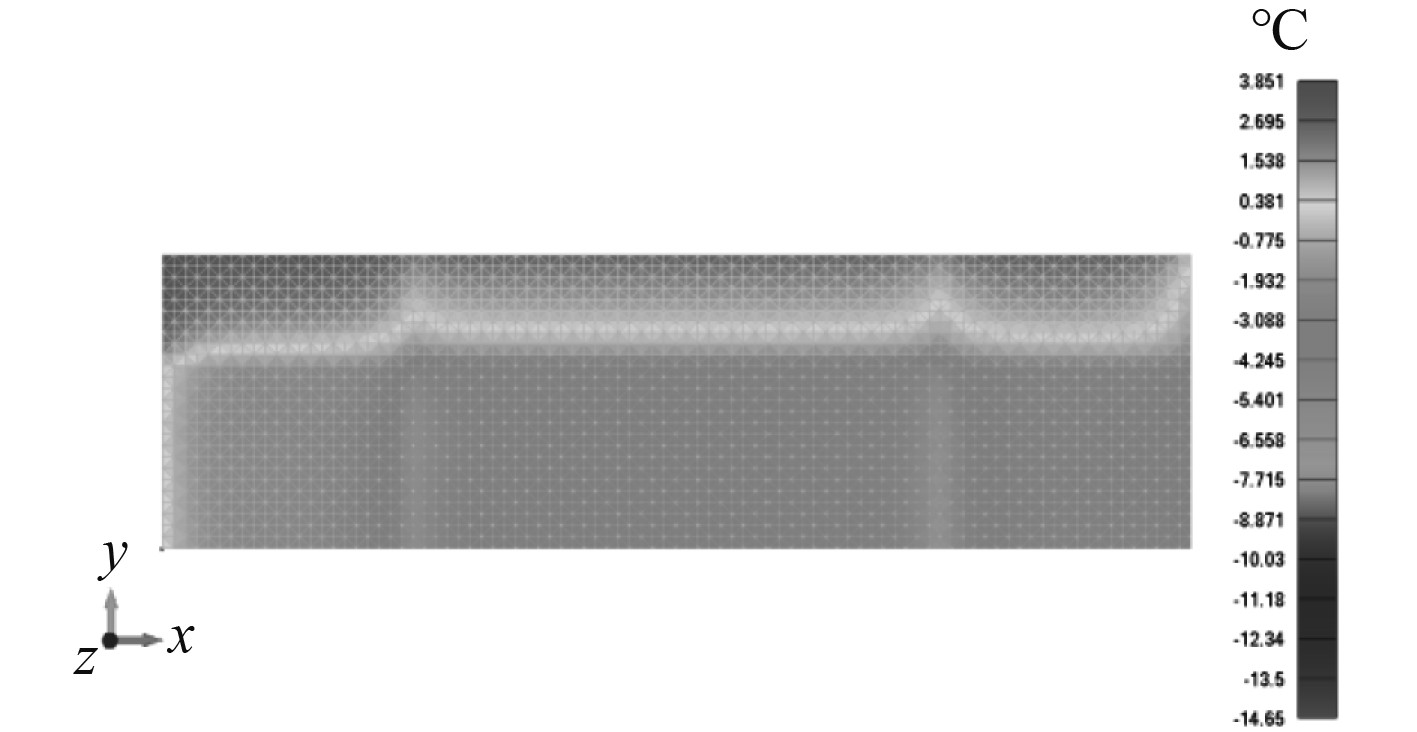图 13 上甲板结构温度场 Fig. 13 Hull structure temperature field of upper deck area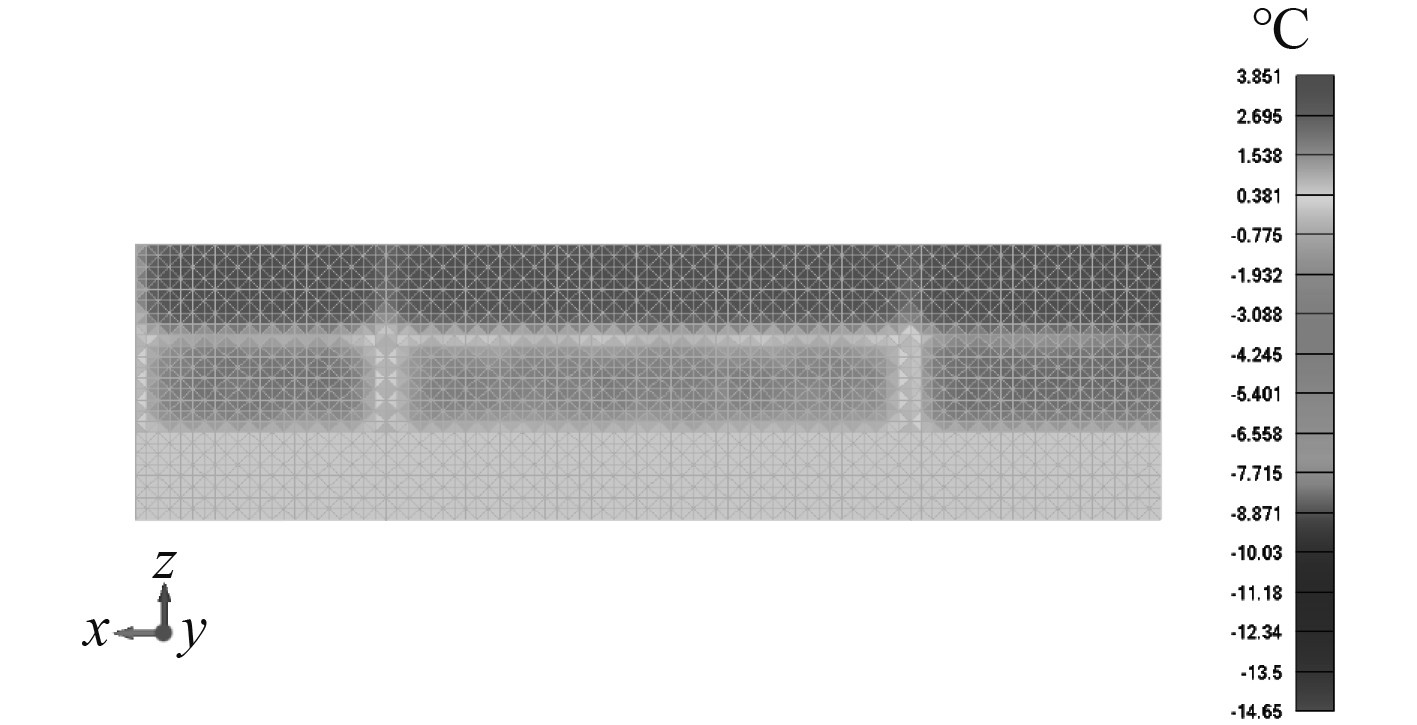图 14 舷侧结构温度场 Fig. 14 Hull structure temperature field of side region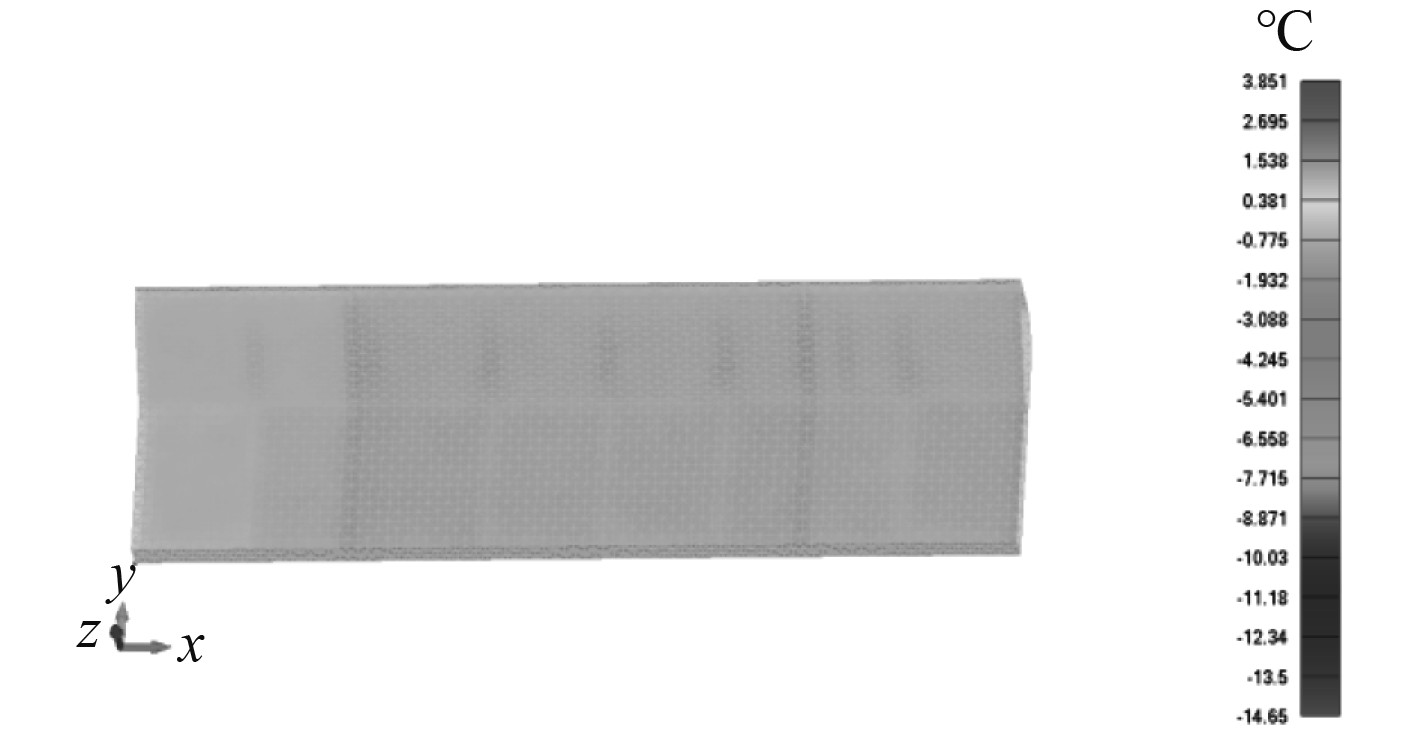图 15 内底及底边舱斜板温度场 Fig. 15 Hull structure temperature filed of inner bottom and hopper
3.2 热阻网络法计算结果图 16 TRNM货舱段结构温度场 Fig. 16 Hull structure temperature field of cargo hold area by TRNM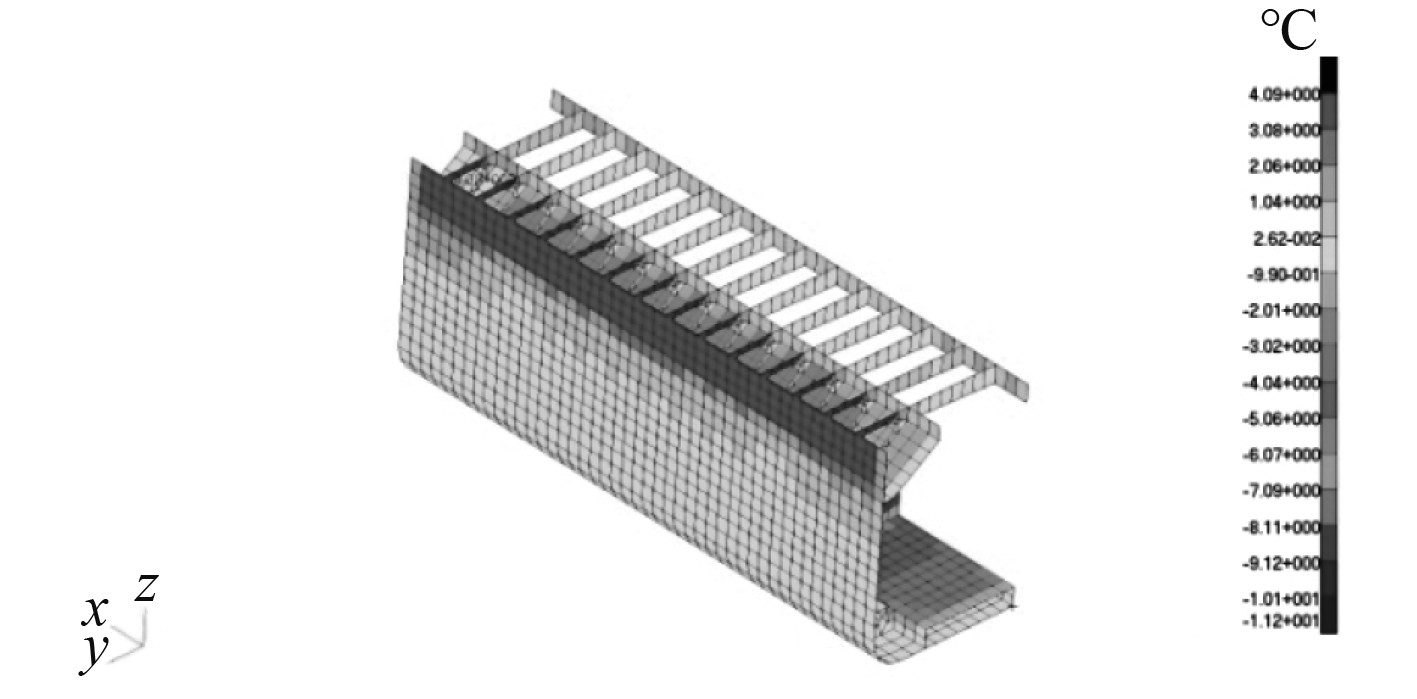图 17 TRNM甲板板架和舷侧温度场 Fig. 17 Hull structure temperature field of deck grillage and side shell by TRNM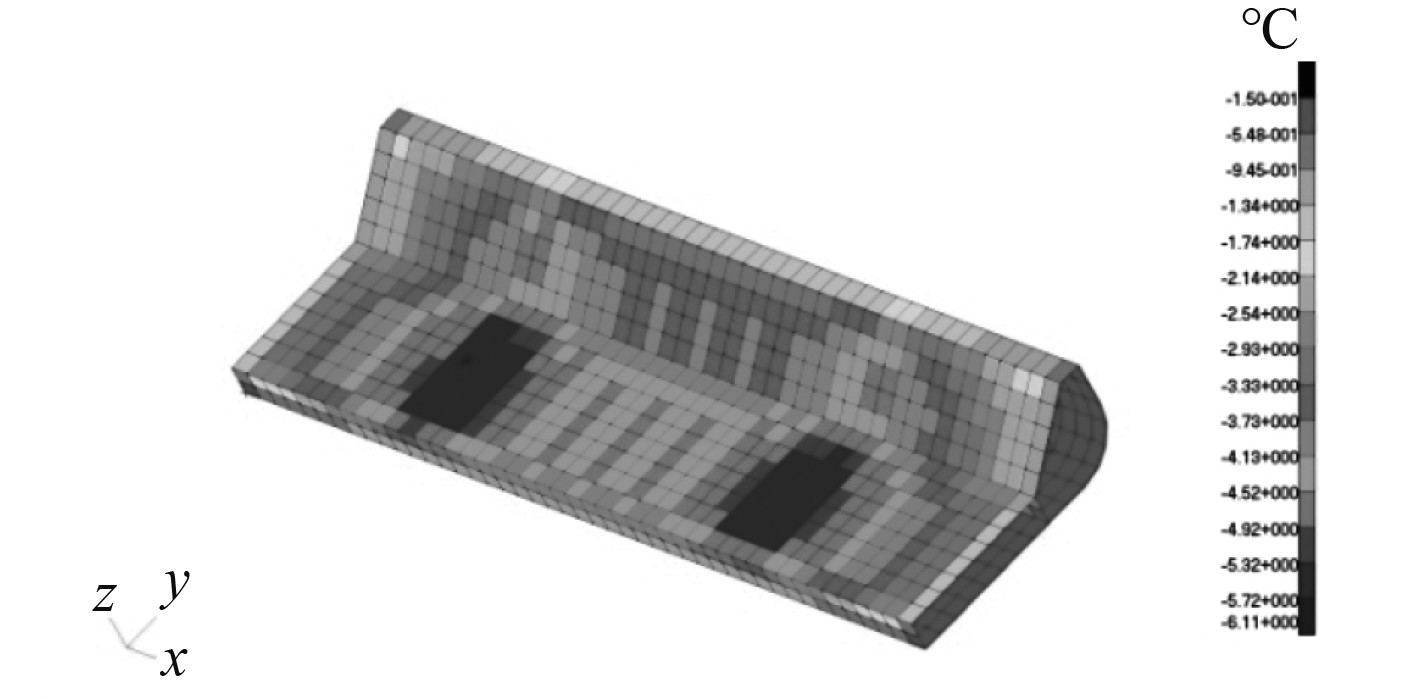图 18 TRNM内底和底边舱斜板温度场 Fig. 18 Hull structure temperature field of inner bottom and hopper by TRNM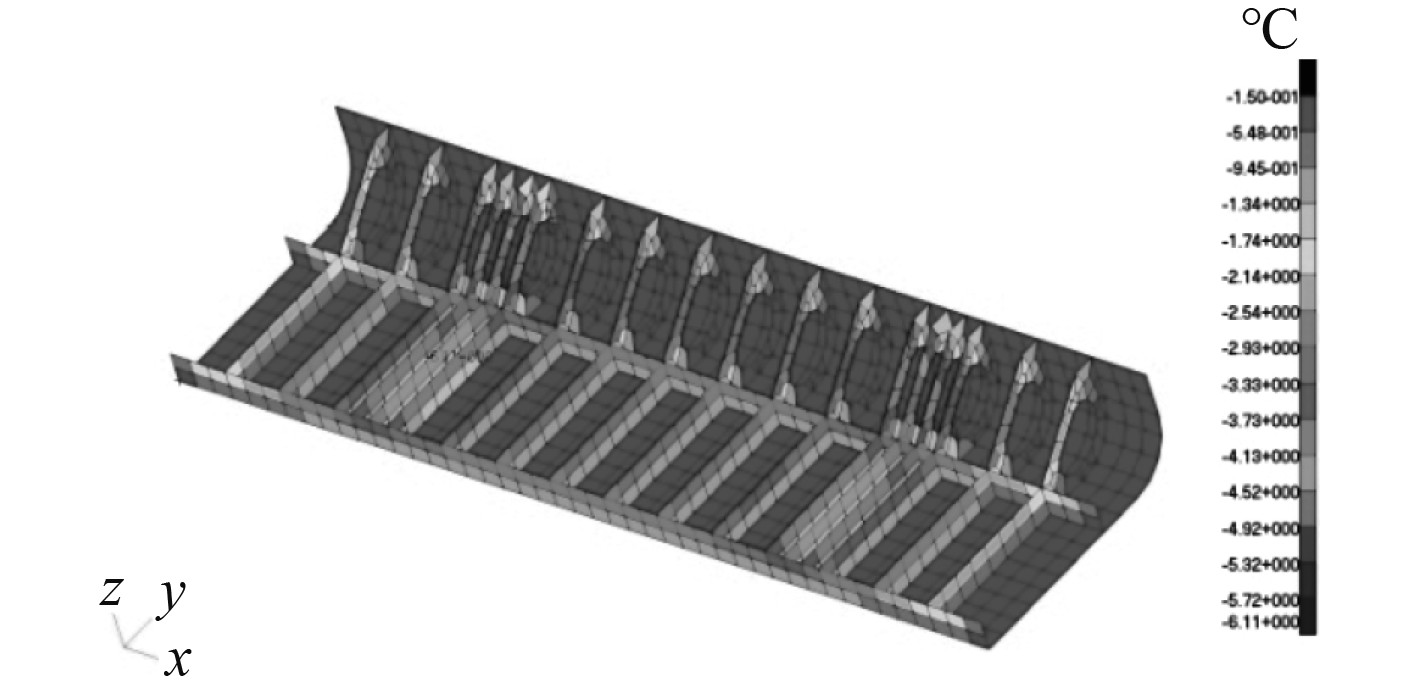图 19 TRNM底部强框温度场 Fig. 19 Hull structure temperature field of bottom floor and web by TRNM
4 鞍座区域结构热阻网络法温度场计算与热应力计算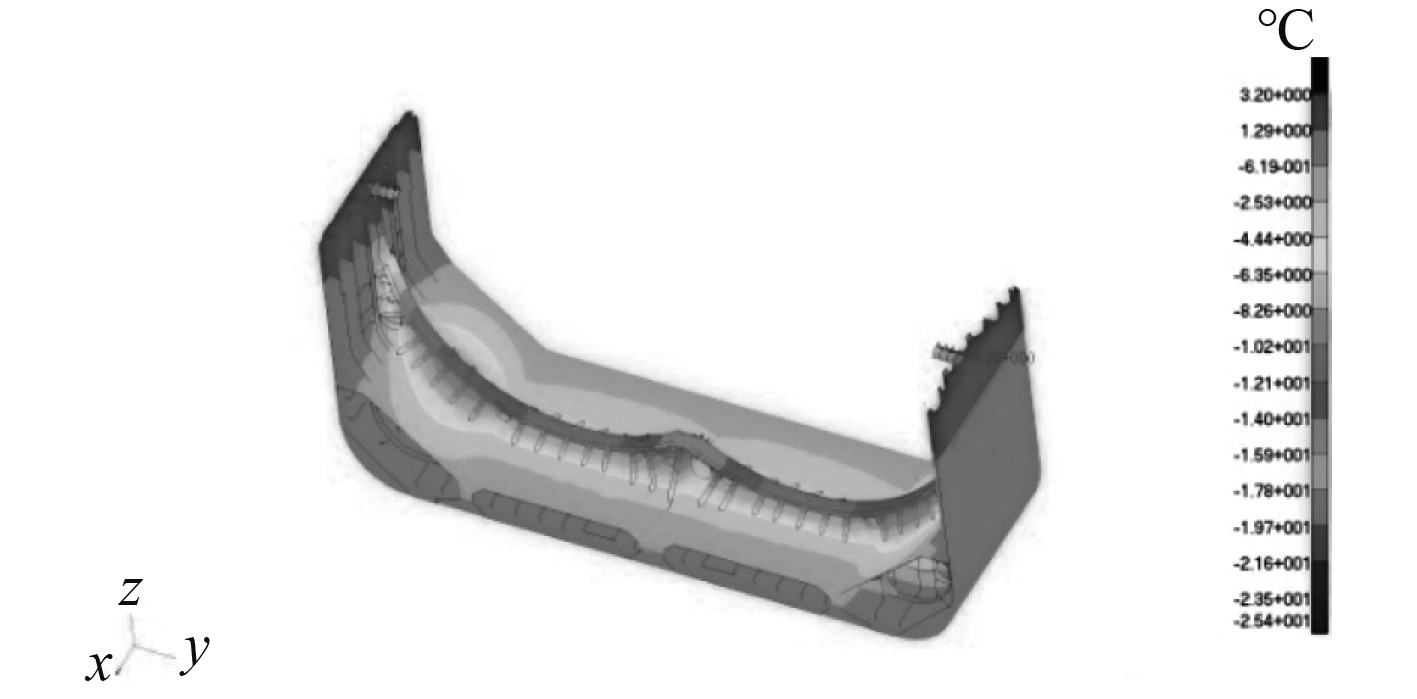图 20 鞍座结构温度分布 Fig. 20 Temperature distribution of saddle structure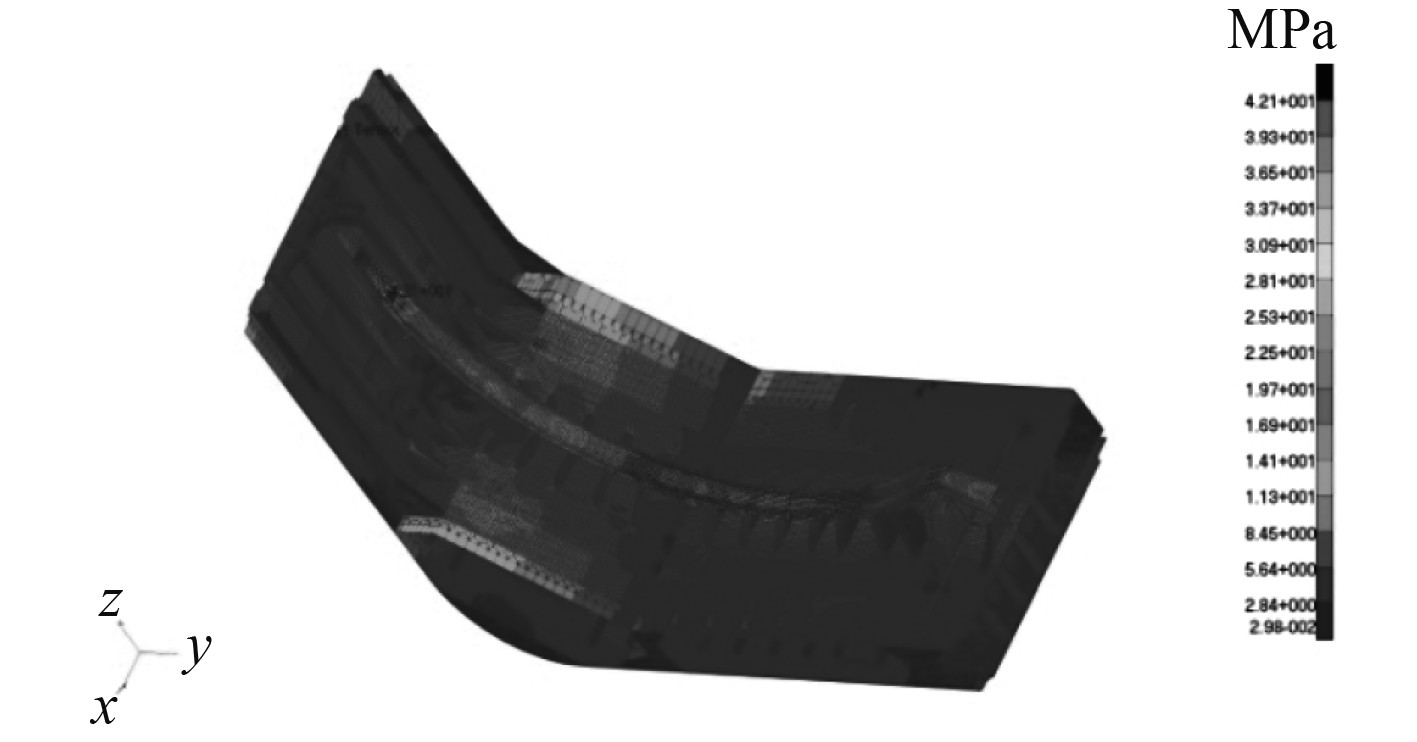图 21 鞍座结构热应力(Von mises应力)分布 Fig. 21 Thermal stress distribution of saddle structure (Von mises stress)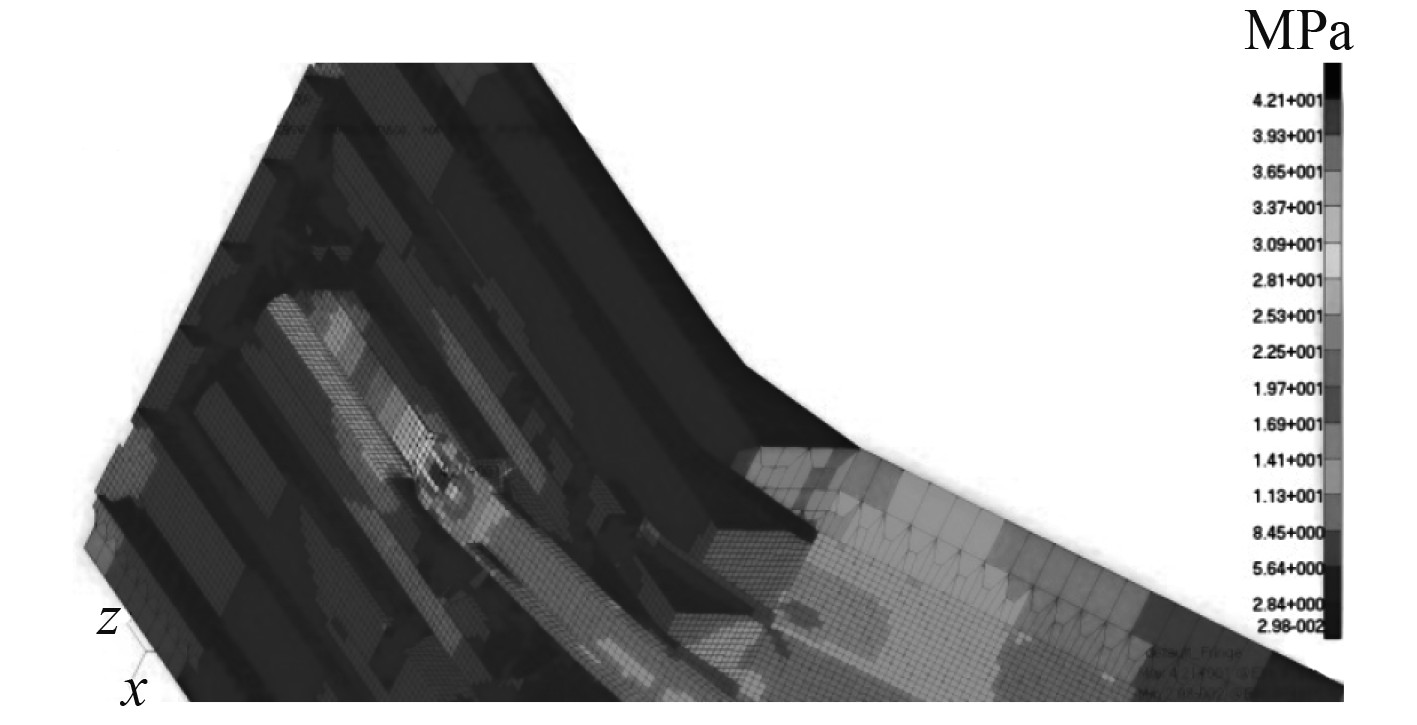图 22 温度梯度较大区域热应力（Von mises应力） Fig. 22 Thermal stress of high temperature gradient region (Von mises stress）
5 结　语

1）本文提出的温度场计算方法将CFD方法和热阻网络法的优势相结合，避免了单独使用CFD方法难以考虑结构细节的缺点以及单独使用热阻网络法较难考虑对流热交换的缺点，为船体结构温度场计算提供了一种实用可靠的办法。

2）实船计算表明，通过CFD方法得到流场速度与温度分布可以为热阻网络法提供合理准确的计算输入，而热阻网络法可以对CFD方法的计算结果进行校核，提高结果的可靠性。

3）实船计算表明，对于关键区域的结构强度评估，应视温度梯度分布进行热应力计算，提高结构的安全性。

4）本文方法可以推广应用到其他类型的液化气船以及双燃料船舶的结构温度场计算中。

  American Bureau of Shipping. Guidance notes on thermal analysis of vessels with tanks for liquefied gas[S]. 2019.  中国船级社. 散装运输液化气体船舶构造与设备规范[S]. 2018.  李小灵, 谷运飞. 计及热辐射及翼翅效应的VLGC温度场计算[J]. 船舶与海洋工程, 2013, 94(2): 15-22. DOI:10.3969/j.issn.2095-4069.2013.02.004  吕立伟, 吴嘉蒙, 余勇华. 薄膜型LNG船横向隔离舱温度场分析的简化算法[J]. 船舶, 2014, 147(3): 20-25. DOI:10.3969/j.issn.1001-9855.2014.03.004  蒋军, 车驰东, 陆晟. C型LNG船货舱区温度场分析[J]. 船舶与海洋工程, 2018, 34(5): 9-14. DOI:10.14056/j.cnki.naoe.2018.05.002  DING Shi-feng, TANG Wen-yong, ZHANG Sheng-kun. Research on temperature field and thermal stress of liquefied natural gas carrier with incomplete insulation[J]. Shanghai Jiaotong University (science) 2010, 15(3): 346-353.  高雯雯. LNG加注船C型舱的温度场研究[D]. 上海: 上海交通大学, 2018.  傅允准, 祁亮, 巨永林, 等. B型独立LNG液货舱漏热量及蒸发率影响因素模拟分析[J]. 船舶工程, 2015, 37(4): 12-16. DOI:10.13788/j.cnki.cbgc.2015.04.012  VERDI J R. 工程传热学[M]. 北京: 人民教育出版社, 1982.  RAJ P. CHHABRA. CRC handbook of thermal engineering Second Edition[M]. New York: CRC Press, 2017.  JOHN D. ANDERSON, JR. 计算流体力学入门[M]. 北京: 清华大学出版社, 2010.  宋慧凯. 应用Sinda及TMG的星载机构热设计和分析方法研究[D]. 哈尔滨: 哈尔滨工业大学, 2012.  IMO. IGC Code: International code for the construction and equipment of ships carrying liquefied gases in bulk[S]. 2016.  陈哲超, 王伟飞, 张志康, 等. LEG船C型舱液罐鞍座设计研究[J]. 舰船科学技术, 2020, 42(4): 65-69. DOI:10.3404/j.issn.1672-7649.2020.04.013